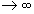The Sum of A Geometric Series of Lengths.

Theorem X.B.1 (Convergence of the fundamental geometric series.) Suppose S n  = 1 + r + r2 + r 3 + ... + r n  . Then

limS n = 1/(1-r) if and only if | r | < 1.

In the figure below, consider the left  most square to have side of length 1. By considering the similar triangles notice that AB/1 = 1/(1-r). But the sides of the squares accumulate to give AB = 1 + r + r2 + r 3 + ... .